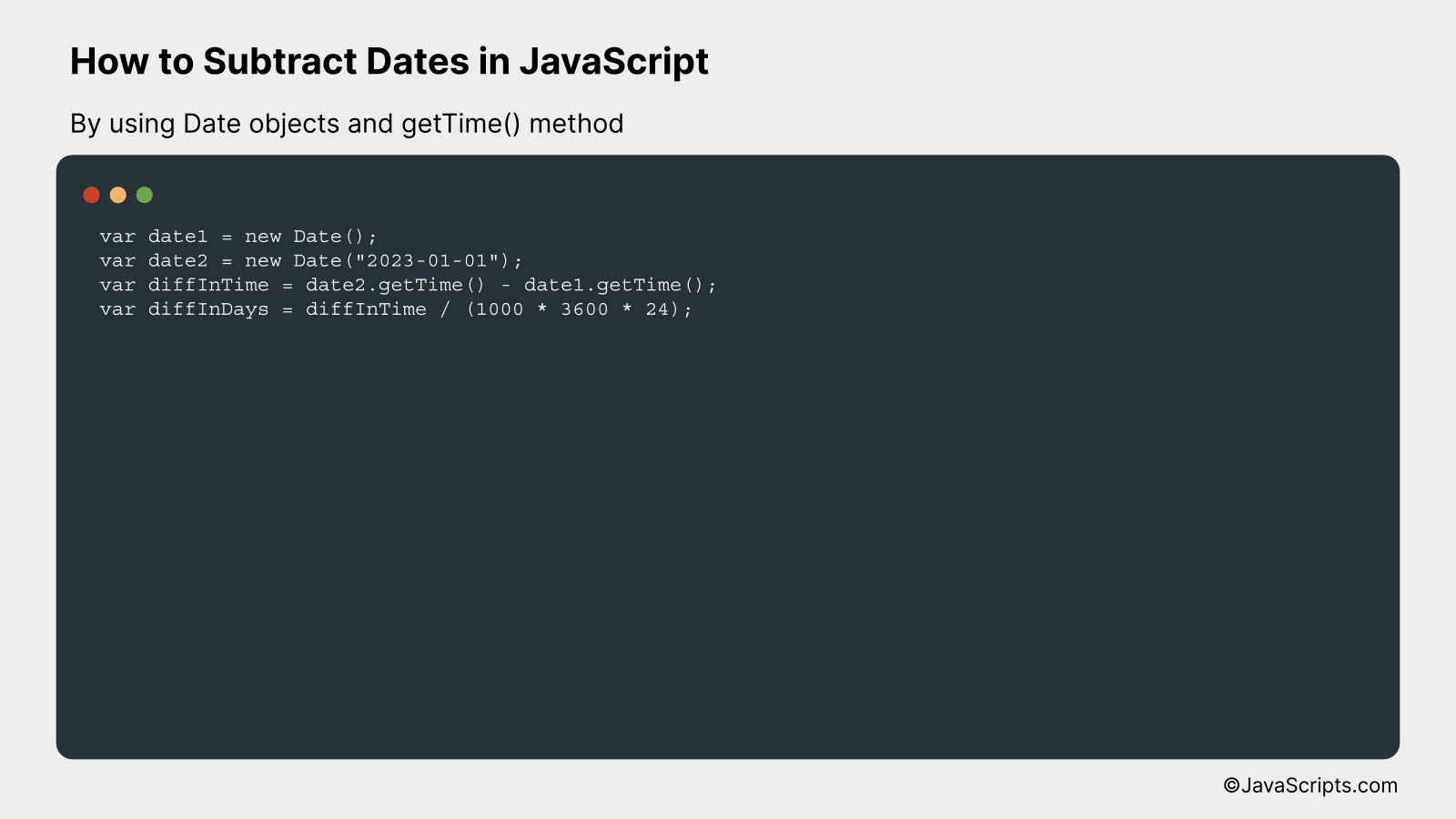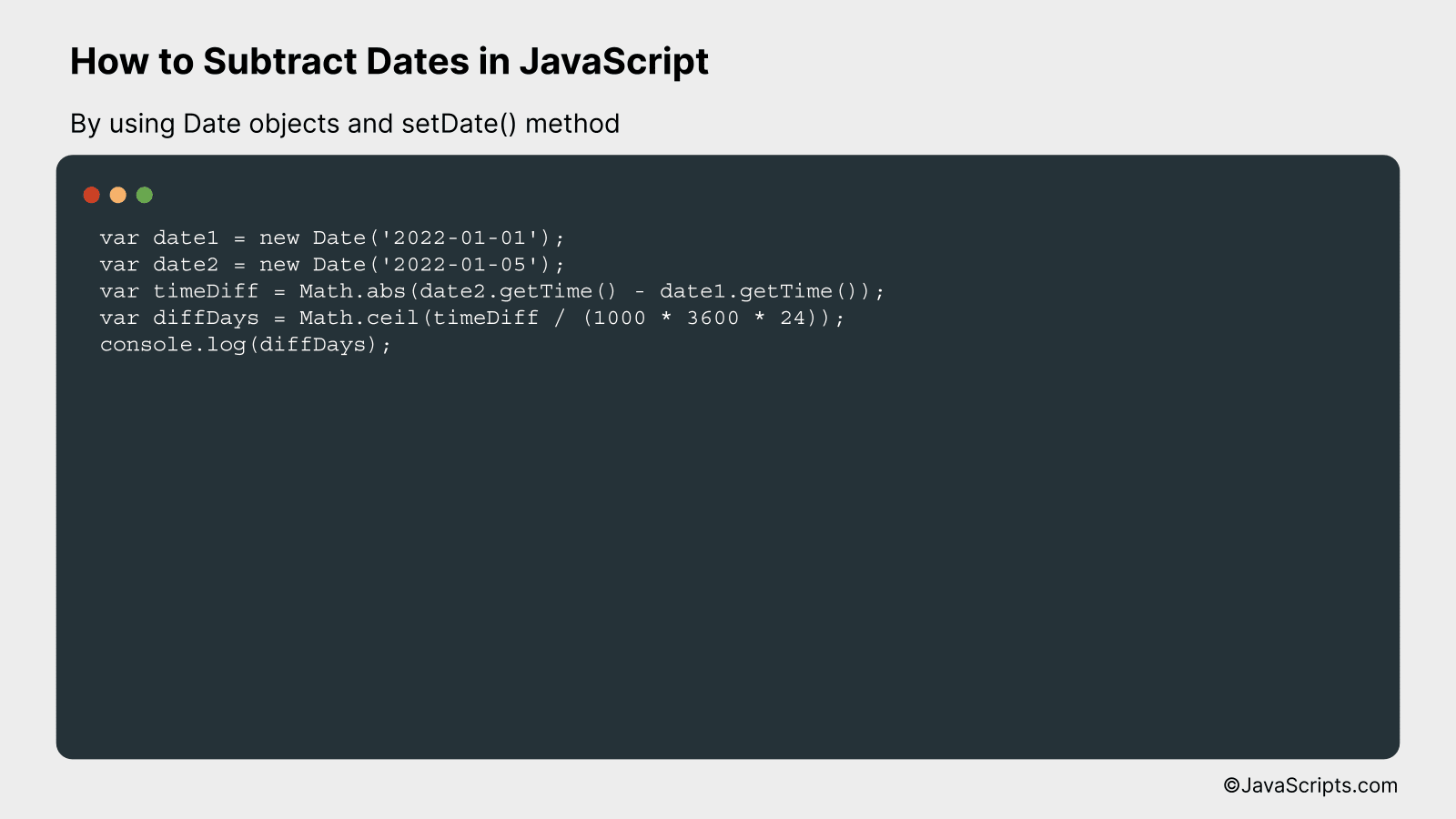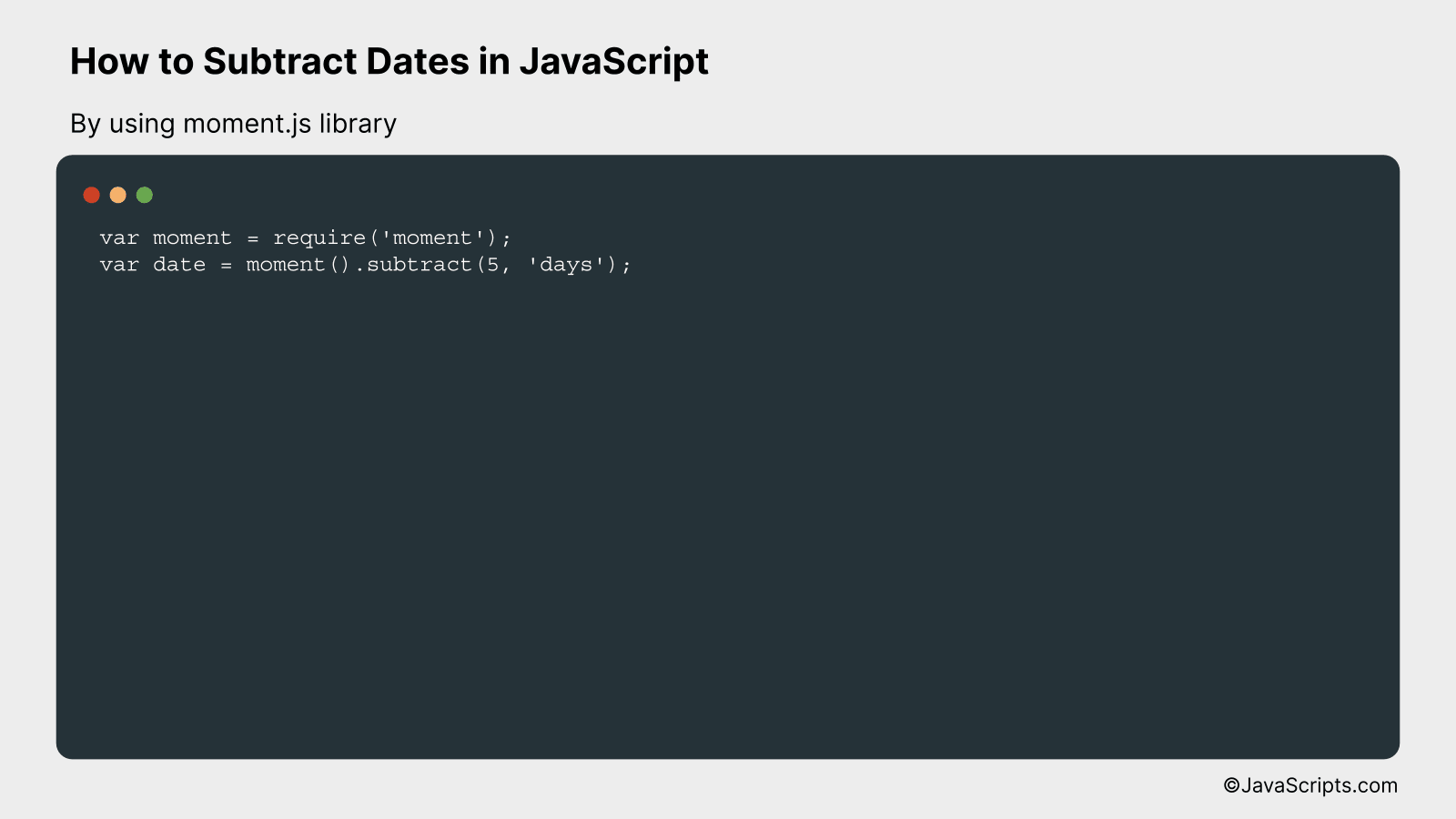# How to Subtract Dates in JavaScript

Navigating the world of JavaScript can sometimes feel like a journey through a dense forest, especially when it comes to handling dates and times. But worry not, together we’ll tackle a common task: subtracting dates.

Isn’t it fascinating how a few lines of code can calculate the time elapsed between two moments? Today, we’ll explore, step by step, the methods for subtracting dates in JavaScript. It’s simpler than you might think!

In this post, we will be looking at the following 3 ways to subtract dates in JavaScript:

• By using Date objects and getTime() method
• By using Date objects and setDate() method
• By using moment.js library

Let’s explore each…

## #1 – By using Date objects and getTime() methodWe will calculate the difference between two dates in JavaScript by subtracting the timestamps (obtained using the getTime() method) of the two Date objects. The result will be in milliseconds, which we will then convert into the desired units (seconds, minutes, hours, days, etc.).

For instance, if we want to calculate the difference between today’s date and January 1, 2023, we’ll instantiate two Date objects for these two dates, convert them into timestamps by calling getTime() method, subtract one timestamp from the other, and finally convert the result from milliseconds into days.

``````
var date1 = new Date();
var date2 = new Date("2023-01-01");
var diffInTime = date2.getTime() - date1.getTime();
var diffInDays = diffInTime / (1000 * 3600 * 24);
``````

### How it works

The JavaScript Date object represents a date and time down to milliseconds. The getTime() method of the Date object returns the number of milliseconds since the Unix epoch (January 1, 1970, 00:00:00 UTC). So by subtracting the timestamp of one date from another, we get the difference in milliseconds. We then convert this to days by dividing by the number of milliseconds in a day (1000 milliseconds/second * 3600 seconds/hour * 24 hours/day).

• The Date() constructor is used to create Date objects. Without arguments, it creates an object for the current date and time. With a date string argument, it creates a Date object for the date specified by the string.
• The getTime() method is called on the Date objects, which returns the timestamp – the number of milliseconds since the Unix epoch.
• The timestamp of the first date is subtracted from the timestamp of the second date, which gives the difference in milliseconds.
• Finally, the difference in milliseconds is converted into days by dividing by the number of milliseconds in a day.

## #2 – By using Date objects and setDate() methodWe will create two JavaScript Date objects, subtract them to get the difference in milliseconds, and then convert these milliseconds into days. This will allow us to calculate the difference between two dates.

``````
var date1 = new Date('2022-01-01');
var date2 = new Date('2022-01-05');
var timeDiff = Math.abs(date2.getTime() - date1.getTime());
var diffDays = Math.ceil(timeDiff / (1000 * 3600 * 24));
console.log(diffDays);
```
```

### How it works

The provided Javascript code calculates the difference in days between two dates by utilizing the Date objects’ getTime() method, which retrieves the timestamp of the date in milliseconds since 1970/01/01, and the Math.abs() method to ensure the result is always positive.

• Step 1: Two Date objects are created with the ‘new’ keyword, named ‘date1’ and ‘date2’, initialized with the dates ‘2022-01-01’ and ‘2022-01-05’ respectively.
• Step 2: The difference in time between ‘date2’ and ‘date1’ is calculated in milliseconds using the getTime() method and stored in the variable ‘timeDiff’. The Math.abs() method is used to ensure that the result is non-negative, which means we always get the absolute difference regardless of the order of dates.
• Step 3: The ‘timeDiff’ is then divided by the number of milliseconds in a day (1000 milliseconds * 3600 seconds * 24 hours) and the result is stored in the variable ‘diffDays’.
• Step 4: Finally, ‘diffDays’ is printed to the console, which represents the difference in days between ‘date1’ and ‘date2’.

## #3 – By using moment.js libraryMoment.js makes it simple to perform mathematical operations like subtracting dates. You can subtract days, months, years, etc from a date. For example, to subtract 5 days from the current date, you can use the `subtract` function of moment.js.

``````
var moment = require('moment');
var date = moment().subtract(5, 'days');
```
```

### How it works

Moment.js has a function named ‘subtract’ which is used to subtract a number of time units from a moment. ‘subtract’ takes two arguments. The first argument is the number of time units to subtract and the second argument is the type of time unit.

• First, we load the moment.js library using the `require` function.
• Then, we use the `moment()` function to get the current date and time.
• Next, we call the `subtract` function on the result of `moment()`, and pass two arguments to it. The first argument is the number of time units we want to subtract, and the second argument is the type of time unit. In our case, we are subtracting 5 days.
• The final result is a moment that is 5 days before the current date and time.

Related:

In conclusion, manipulating dates in JavaScript is a simple yet powerful skill. The Date object is a handy tool that, once mastered, can help you efficiently subtract dates and perform other date-related operations.

Remember, to subtract dates, you simply convert them into milliseconds, perform the subtraction, and convert back to the desired format. This process is straightforward and effective for various applications. Keep practicing, and you’ll get the hang of it in no time.